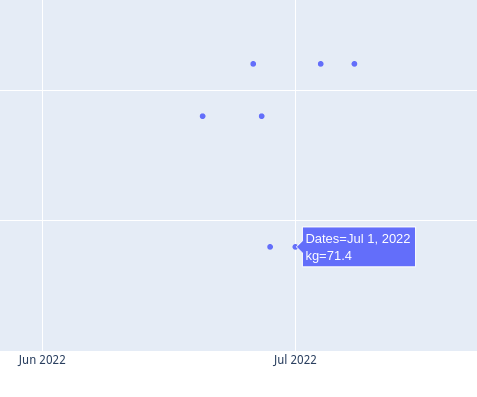# Track my weight using Python

Last edited on 2022-10-13 Tagged under  #python   #programming

I maintain a text file that serves as my "daily log" where I enter various things: observations, things I track over time, things to do, mini HOWTOs, etc.

One item of interest I've been recording is my weight. Each entry includes the date, a `:weight:` tag, and the measurement (in kg).

A sample entry looks like ...

``````2022-07-08T07:19
:weight: 72.1
``````

After several years of weigh-ins and recordings, I've accumulated 1,200+ data points. How can I retrieve these measurements that are mixed in with all other sorts of data?

Enter the power of Python!

I divide this challenge into two parts: 1. Create a Python module (tracktivity) with functions to track any sort of activity (not just weight) in my daily log, and 2. write the actual weight tracking program itself (track_weight.py).

## Tracktivity

I make use of Python regular expressions to scan a text file for matching date and value regex patterns of interest, and create a dictionary of the dates and values found as key-value pairs. I can choose to display the results as a dictionary, formatted into columns with summary, or as a scatter plot graph courtesy of plotly.express.

Module `re` is included in Python. Install `plotly` via `pip` ...

``````\$ pip install plotly
``````

Import the necessary modules ...

``````import re
import plotly.express as px
``````

Function `date_value_dict` scans a text file for date regex with a corresponding value regex match, and returns a dictionary of dates and values as key-value pairs ...

``````def date_value_dict(textfile, date_regex, value_regex, value_matchgroup=0):
dates_values = {}

with open(textfile) as file_obj:
current_date = ""  # most recent date match
for line in file_obj:
if date := re.search(date_regex, line):
current_date = date.group()
if value := re.search(value_regex, line):
try:
value = float(value.group(value_matchgroup))
except ValueError:
value = value.group(value_matchgroup)
dates_values[current_date] = value
return dates_values
``````

Function `date_value_columns` takes a dictionary and returns a list of tuples (formatted using a template), each containing (item number, date string, value) ...

``````def date_value_columns(dictionary, template):
dates_values = [template.format(i, k, v) for i, (k, v) in enumerate(dictionary.items())]
return dates_values
``````

Function `date_value_summary` takes a dictionary of dates and values, and returns a list summary ...

``````def date_value_summary(dictionary):
dct = dictionary
items = len(dct)
summary = [
f"\nLatest:\t{next(iter(dct.items()))}\t(recorded on {next(iter(dct.items()))})",
f"High:\t{max(dct.values())}\t(recorded on {max(dct, key=dct.get)})",
f"Low:\t{min(dct.values())}\t(recorded on {min(dct, key=dct.get)})",
f"Mean:\t{(sum(dct.values()) / items):.1f}\t(calculated from {items} total items)",
]
return summary
``````

Function `date_value_scatterplot` takes a dictionary of dates and values as key-value pairs, and returns a scatter plot graph with title and label for dates (x-axis) and values (y-axis) ...

``````def date_value_scatterplot(dictionary, title, label="Values"):
x_dates = list(dictionary.keys())
y_values = list(dictionary.values())
x_dates.reverse()
y_values.reverse()

fig = px.scatter(x=x_dates, y=y_values, title=title, labels={"x": "Dates", "y": label})
fig.show()
``````

## track_weight.py

I create `track_weight.py` that uses the tracking functions from the `tracktivity` module to scan my daily log for weight measurements and dates recorded, for the purpose of tracking my weight over time.

With the Python module `argparse`, I can add command-line options to my program ...

``````#!/usr/bin/env python3
import argparse
import tracktivity as track
``````

Function `get_args` collects the command-line arguments: `-h` and `--help` for a description of the program; `-r` and `--results` to display output as a `dictionary`, as neatly formatted `columns`, or a `scatterplot` opened in a web browser (default is `columns` if no argument provided) ...

``````def get_args():
parser = argparse.ArgumentParser(
description="""
Scan my daily log for weight measurements and dates recorded for the purpose of tracking my weight over time.
""",
formatter_class=argparse.ArgumentDefaultsHelpFormatter,
)
"-r",
"--results",
metavar="type",
type=str,
choices=["dictionary", "columns"],
default="columns",
help="Display results as: dictionary, columns",
)
return parser.parse_args()
``````

Variables ...

``````args = get_args()
results = args.results
logfile = "/home/dwa/doc/wiki/index.wiki"  # data source
date = r"^20\d\d-\d\d-\d\d"  # find date pattern at beginning of line
value = r"^(:weight:)\s(\d\d.\d)"  # parentheses create groups in the regex for the group() match obj method
matchgroup = 2  # part of value, enclosed in parentheses, to match; 0 = match entire regex
title = "Track Weight (kg)"
label = "kg"
template = "{0:5}  {1:11} {2:4}"  # three-column layout with padding
``````

Create the dictionary of dates and weights ...

``````dates_values = track.date_value_dict(logfile, date, value, matchgroup)
``````

Choose how to display the results ...

``````if results == "dictionary":
print(dates_values)
elif results == "scatterplot":
track.date_value_scatterplot(dates_values, title, label)
elif results == "columns":
print(f"\n{title}\n" + "-" * len(title) + "\n" + template.format("Items", "Dates", "Values"))
print("\n".join(track.date_value_columns(dates_values, template)))
print("\n".join(track.date_value_summary(dates_values)))
``````

Now, if I run `track_weight.py` with `--results dictionary` I get all my weigh-in measurements and the dates they were recorded as a Python dictionary ...

``````\$ track_weight.py --results dictionary
{'2022-07-08': 72.1, '2022-07-04': 72.1, '2022-07-01': 71.4, ...}
``````

With the data stored in a dictionary, its easy to use it in other functions that can work with and present the data in different ways.

Here I arrange the data into columns (the default), with a summary at the end ...

``````\$ track_weight.py

Track Weight (kg)
-----------------
Items  Dates       Values
0  2022-07-08  72.1
1  2022-07-04  72.1

[...]

1232  2014-01-19  77.6
1233  2014-01-12  79.8

Latest:	72.1	(recorded on 2022-07-08)
High:	79.8	(recorded on 2014-01-12)
Low:	59.8	(recorded on 2014-12-12)
Mean:	66.9	(calculated from 1234 total items)
``````

To better appreciate the changes over time, its helpful to visualize the data as a scatter plot ...

``````\$ track_weight.py -r scatterplot
``````

A graph is generated by `plotly.express`, and `fig.show()` opens an interactive graph in a web browser ...I really enjoyed putting this together as my first Python data analysis project. Working on a project of personal interest, something that you regularly use, is the very best way to learn how to program (or anything for that matter)!

Source: tracktivity